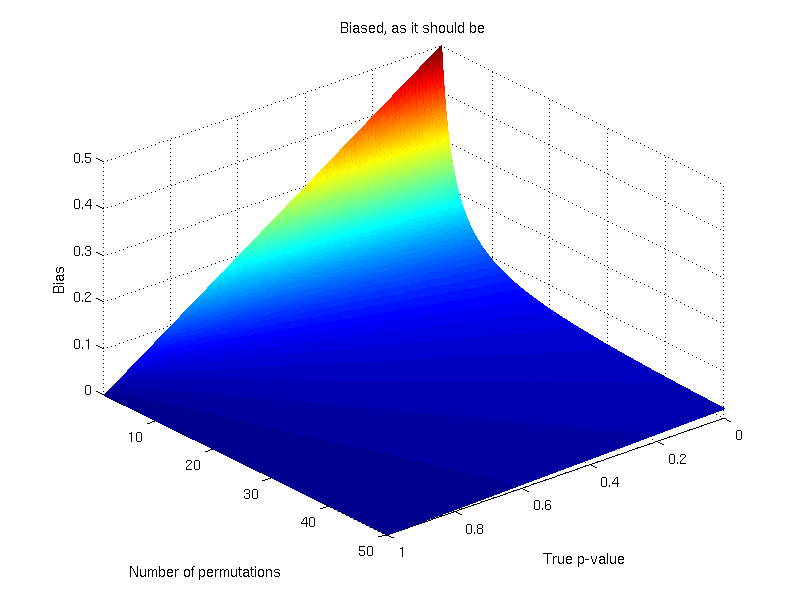# Biased, as it should beWhen we run a permutation test, what we do is to first compute the statistic for the unpermuted model, then shuffle the data randomly, compute a new statistic and, if it is larger than the statistic for the unpermuted model, increment a counter. We then repeat the random shuffling, and keep incrementing the counter until we think we are done with them. We then divide the counter by the number of permutations and the result is the p-value what we wanted to find.

This procedure can be stated as:$\hat{p} = \frac{1}{N}\sum^{N}_{n=1}\mathcal{I}(T^{*}_{n} \geqslant T_0)$

where$\hat{p}$ is the estimated p-value,$N$ is the number of permutations,$T_0$ is the statistic for the unpermuted model,$T^{*}_n$ is the statistic for the$n$-th random shuffling, and$\mathcal{I}$ is an indicator function that evaluates as 1 if the condition between parenthesis is true, or 0 otherwise. This formulation produces unbiased results: since$E(\mathcal{I}(T^{*}_{n} \geqslant T_0)) = p$, the true p-value, then$E(\frac{1}{N}\sum^{N}_{n=1}\mathcal{I}(T^{*}_{n} \geqslant T_0)) = p$.

## The problem

This strategy, however, has a problem. If the true p-value,$p$, is small, or if the number of permutations is not sufficiently large, even after all the$N$ permutations are done, no$T^{*}_{n}$ may reach or surpass the value of$T_0$, resulting in a p-value equal to 0. While this is still a valid, unbiased result, p-values equal to zero are problematic, as they can be interpreted as indicating the rejection of the null-hypothesis with absolute confidence. And this even after very few permutations (perhaps just 1!) have been performed, which is indeed rather counter-intuitive. We cannot be completely sure of the rejection of the null simply for having done just a few permutations.

## Biasing to solve the problem

The solution is to make two modifications to the formulation above: start counting the$n$-th permutation at 0, for the unpermuted model, and divide the counter at the end not by$N$, but by$N+1$:$\hat{p} = \frac{1}{N+1}\sum^{N}_{n=0}\mathcal{I}(T^{*}_{n} \geqslant T_0)$

This formulation, which pools the unpermuted model together with the permuted realisations, is biased: for$n=0$,$\mathcal{I}(T^{*}_{n} \geqslant T_0) = 1$,  and so,$E(\frac{1}{N+1}\sum^{N}_{n=0}\mathcal{I}(T^{*}_{n} \geqslant T_0)) = \frac{1+Np}{N+1} \neq p$.

## Quantifying the bias

The bias is smaller for a large number of permutations, and converges to zero as$N$ increases towards infinity. What is important, however, is that the bias punishes the results towards conservativeness whenever the true and unknown$p$ is smaller than$\frac{1}{N+1}$, in a way that no p-value smaller than$\frac{1}{N+1}$ can ever be attained. It forces the researcher to run more permutations to find such small p-values to reject the null hypothesis, which otherwise could be rejected with a p-value equal to zero that would appear easily even after just a handful of permutations.The bias is larger for smaller number of permutations and for smaller true p-values.

## Controversy

The solution has been proposed by some authors, such as Edgington (1995), and strongly advocated by, e.g., Phipson and Smyth (2010). While the biased solution is definitely the most adequate for practical scientific problems, it can be considered as of little actual statistical meaning. Pesarin and Salmaso (2010) explain that the distribution of$T_0$ is not the same as the distribution of$T^{*}_{n}$, unless the null is true. Under the alternative hypothesis, therefore,$T_0$ should not be pooled into the empirical distribution from which the p-value is obtained. Moreover, under the null, all possible permutations have the same probability, with no intrinsic reason to treat some (as the unpermuted) differently than others. If one wants unbiased results, the alternative formulation should obviously not be used.